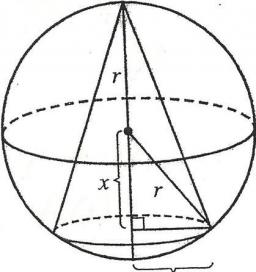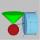# Sphere and cone

Within the sphere of radius G = 33 cm inscribe the cone with the largest volume. What is that volume, and what are the dimensions of the cone?

r =  31.11 cm
h =  44 cm
V =  44602.24 cm3

### Step-by-step explanation:

Our quadratic equation calculator calculates it.Did you find an error or inaccuracy? Feel free to write us. Thank you!

Showing 1 comment:Dr Math
that's very mind blowingTips to related online calculators
Looking for help with calculating roots of a quadratic equation?
Pythagorean theorem is the base for the right triangle calculator.

#### You need to know the following knowledge to solve this word math problem:

We encourage you to watch this tutorial video on this math problem:

## Related math problems and questions:

• Sphere in coneA sphere of radius 3 cm describes a cone with minimum volume. Determine cone dimensions.
• ConeInto rotating cone with dimensions r = 8 cm and h = 8 cm incribe cylinder with maximum volume so that the cylinder axis is perpendicular to the axis of the cone. Determine the dimensions of the cylinder.
• Paper boxThe hard rectangular paper has dimensions of 60 cm and 28 cm. The corners are cut off equal squares, and the residue was bent to form an open box. How long must beside the squares be the largest volume of the box?
• Cube in sphereThe cube is inscribed in a sphere with a radius r = 6 cm. What percentage is the volume of the cube from the volume of the ball?
• Secret treasureScouts have a tent in the shape of a regular quadrilateral pyramid with a side of the base 4 m and a height of 3 m. Find the container's radius r (and height h) so that they can hide the largest possible treasure.
• Cube in sphereThe sphere is an inscribed cube with an edge of 8 cm. Find the sphere's radius.
• CuboidCuboid with edge a=6 cm and space diagonal u=31 cm has volume V=900 cm3. Calculate the length of the other edges.
• Body diagonalCalculate the length of the body diagonal of a block with dimensions: a = 20 cm, b = 30 cm, c = 15 cm.
• Ratio of edgesThe dimensions of the cuboid are in a ratio 3: 1: 2. The body diagonal has a length of 28 cm. Find the volume of a cuboid.
• Tangent spheresA sphere with a radius of 1 m is placed in the corner of the room. What is the largest sphere size that fits into the corner behind it? Additional info: Two spheres are placed in a corner of a room. The spheres are each tangent to the walls and floor and
• Cut and coneCalculate the volume of the rotation cone which lateral surface is circle arc with radius 15 cm and central angle 63 degrees.
• Truncated cone 5The height of a cone 7 cm and the length of side is 10 cm and the lower radius is 3cm. What could the possible answer for the upper radius of truncated cone?
• Two ballsTwo balls, one 8cm in radius and the other 6cm in radius, are placed in a cylindrical plastic container 10cm in radius. Find the volume of water necessary to cover them.
• ConeCalculate the volume of the rotating cone with a base radius 26.3 cm and a side 38.4 cm long.
• Maximum of volumeThe shell of the cone is formed by winding a circular section with a radius of 1. For what central angle of a given circular section will the volume of the resulting cone be maximum?
• Cube and sphereCube with the surface area 150 cm2 is described sphere. What is sphere surface?
• Cuboid - volume, diagonalsThe length of the one base edge of cuboid a is 3 cm. Body diagonal is ut=13 cm and diagonal of cuboid's baseis u1=5 cm. What is the volume of the cuboid?